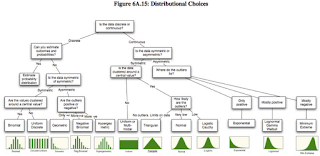# Fitting distributions with R

Fitting distribution with R is something I have to do once in a while, but where do I start?

A good starting point to learn more about distribution fitting with R is Vito Ricci's tutorial on CRAN. I also find the vignettes of the actuar and fitdistrplus package a good read. I haven't looked into the recently published Handbook of fitting statistical distributions with R, by Z. Karian and E.J. Dudewicz, but it might be worthwhile in certain cases, see Xi'An's review. A more comprehensive overview of the various R packages is given by the CRAN Task View: Probability Distributions, maintained by Christophe Dutang.

How do I decide which distribution might be a good starting point?

I came across the paper Probabilistic approaches to risk by Aswath Damodaran. In Appendix 6.1 Aswath discusses the key characteristics of the most common distributions and in Figure 6A.15 he provides a decision tree diagram for choosing a distribution:Figure 6A.15 from Probabilistic approaches to risk by Aswath Damodaran

JD Long points to the Clickable diagram of distribution relationships by John Cook in his blog entry about Fitting distribution X to data from distribution Y . With those two charts I find it not too difficult anymore to find a reasonable starting point.

Once I have decided which distribution might be a good fit I start usually with the fitdistr function of the MASS package. However, since I discovered the fitdistrplus package I have become very fond of the fitdist function, as it comes with a wonderful plot method. It plots an empirical histogram with a theoretical density curve, a QQ and PP-plot and the empirical cumulative distribution with the theoretical distribution. Further, the package provides also goodness of fit tests via gofstat.

Suppose I have only 50 data points, of which I believe that they follow a log-normal distribution. How much variance can I expect? Well, let's experiment. I draw 50 random numbers from a log-normal distribution, fit the distribution to the sample data and repeat the exercise 50 times and plot the results using the plot function of the fitdistrplus package.

I notice quite a big variance in the results. For some samples other distributions, e.g. logistic, could provide a better fit. You might argue that 50 data points is not a lot of data, but in real life it often is, and hence this little example already shows me that fitting a distribution to data is not just about applying an algorithm, but requires a sound understanding of the process which generated the data as well.What is a good book or paper to read about the practical utility of data fitting ? I find it  easier to use R than to get at the use of this. Is this used to compare data sets from different load tests ?

Markus Gesmann said...

I am sorry, but I don't understand your question. The above post points you to some papers which explain distributions fitting in more detail.I was looking at some capacity planning issues and know of various good books. So I was trying to understand what kind of statistical analysis of future workload will result from the effort to find what distribution the data models. I use R. I think I am missing the data analysis part.
it ?I love that decision tree, thanks for posting. What do you recommend doing if you have a column of scores and have no clue what the distribution is? What's steps do you take to determine what it might be? I need to do this to two columns so that I can determine how to determine correlation between them.

Markus Gesmann said...

Plot the data to understand what the distribution looks like.Thanks for your reply Markus. So after I remove outliers from the source data you suggest I do a qq plot and/or a histogram in R first? Then do something like above to confirm what I inferred by the plot?

very helpful article. Thanks a millionanand said...

very nice.. Thanks a lot !anand said...

Hi again,

I am using fitdist function to calculate alpha and beta parameters of gamma distribution. Now when I call plot function on fitdist object, it produces nice plot as you have mentioned in your article. But I want only the emperical and theoritical distr. plot (first one - the histogram with density curved overlapped onto it). How do I get that single plot ??